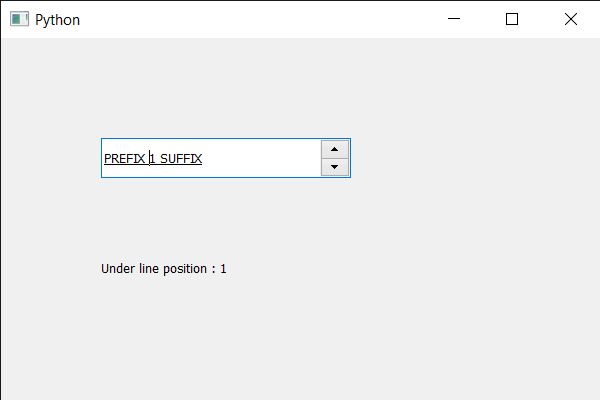Related Articles
PyQt5 QSpinBox – Getting the under line position
• Last Updated : 28 May, 2020

In this article we will see how we can get under line position of the spin box’s text. Under line position is the distance from the base line to where an under line should be drawn. An underline, also called an underscore, is a more or less horizontal line immediately below a portion of writing. Single and occasionally double underlining is used in hand-written or typewritten documents as a way to emphasize key text.

In order to do this we use `strikeOutPos` method with the spin box’s QFontMetrics object.

Syntax : font_metrics.underlinePos()

Argument : It takes no argument

Return : It returns integer.

Below is the implementation

 `# importing libraries``from` `PyQt5.QtWidgets ``import` `*` `from` `PyQt5 ``import` `QtCore, QtGui``from` `PyQt5.QtGui ``import` `*` `from` `PyQt5.QtCore ``import` `*` `import` `sys`` ` ` ` `class` `Window(QMainWindow):`` ` `    ``def` `__init__(``self``):``        ``super``().__init__()`` ` `        ``# setting title``        ``self``.setWindowTitle(``"Python "``)`` ` `        ``# setting geometry``        ``self``.setGeometry(``100``, ``100``, ``600``, ``400``)`` ` `        ``# calling method``        ``self``.UiComponents()`` ` `        ``# showing all the widgets``        ``self``.show()`` ` `        ``# method for widgets``    ``def` `UiComponents(``self``):``        ``# creating spin box``        ``self``.spin ``=` `QSpinBox(``self``)`` ` `        ``# setting geometry to spin box``        ``self``.spin.setGeometry(``100``, ``100``, ``250``, ``40``)`` ` `        ``# setting range to the spin box``        ``self``.spin.setRange(``1``, ``999999``)`` ` `        ``# setting prefix to spin``        ``self``.spin.setPrefix(``"PREFIX "``)`` ` `        ``# setting suffix to spin``        ``self``.spin.setSuffix(``" SUFFIX"``)`` ` `        ``# setting under line to the font``        ``font ``=` `self``.spin.font()``        ``font.setUnderline(``True``)``        ``self``.spin.setFont(font)`` ` `        ``# creating a label``        ``label ``=` `QLabel(``self``)`` ` `        ``# making label multi line``        ``label.setWordWrap(``True``)`` ` `        ``# setting geometry to the label``        ``label.setGeometry(``100``, ``200``, ``300``, ``60``)`` ` `        ``# getting font metrics``        ``f_metrics ``=` `self``.spin.fontMetrics()`` ` `        ``# getting the under line position``        ``value ``=` `f_metrics.underlinePos()`` ` `        ``# setting text to the label``        ``label.setText(``"Under line position : "` `+` `str``(value))`` ` ` ` `# create pyqt5 app``App ``=` `QApplication(sys.argv)`` ` `# create the instance of our Window``window ``=` `Window()`` ` `# start the app``sys.exit(App.``exec``())`

Output :Attention geek! Strengthen your foundations with the Python Programming Foundation Course and learn the basics.

To begin with, your interview preparations Enhance your Data Structures concepts with the Python DS Course.

My Personal Notes arrow_drop_up